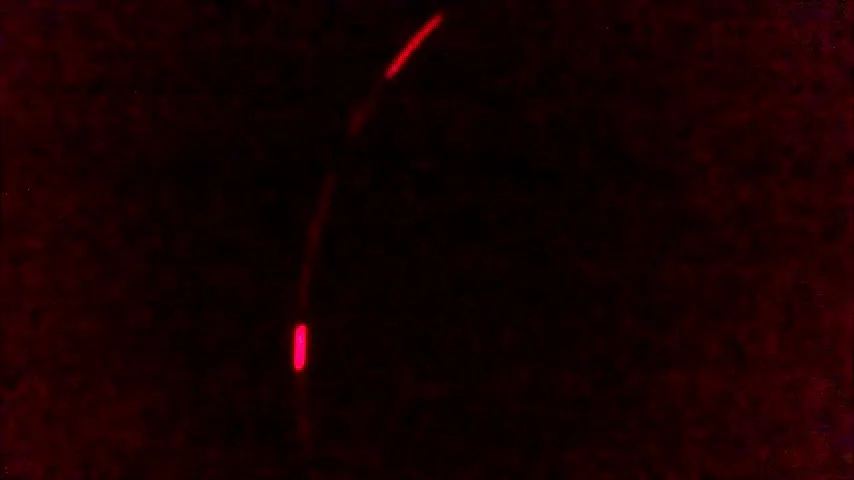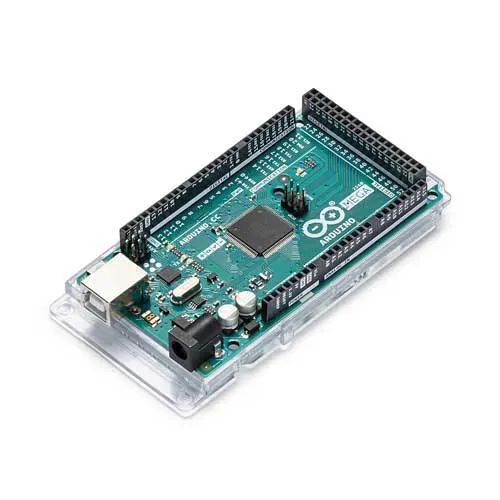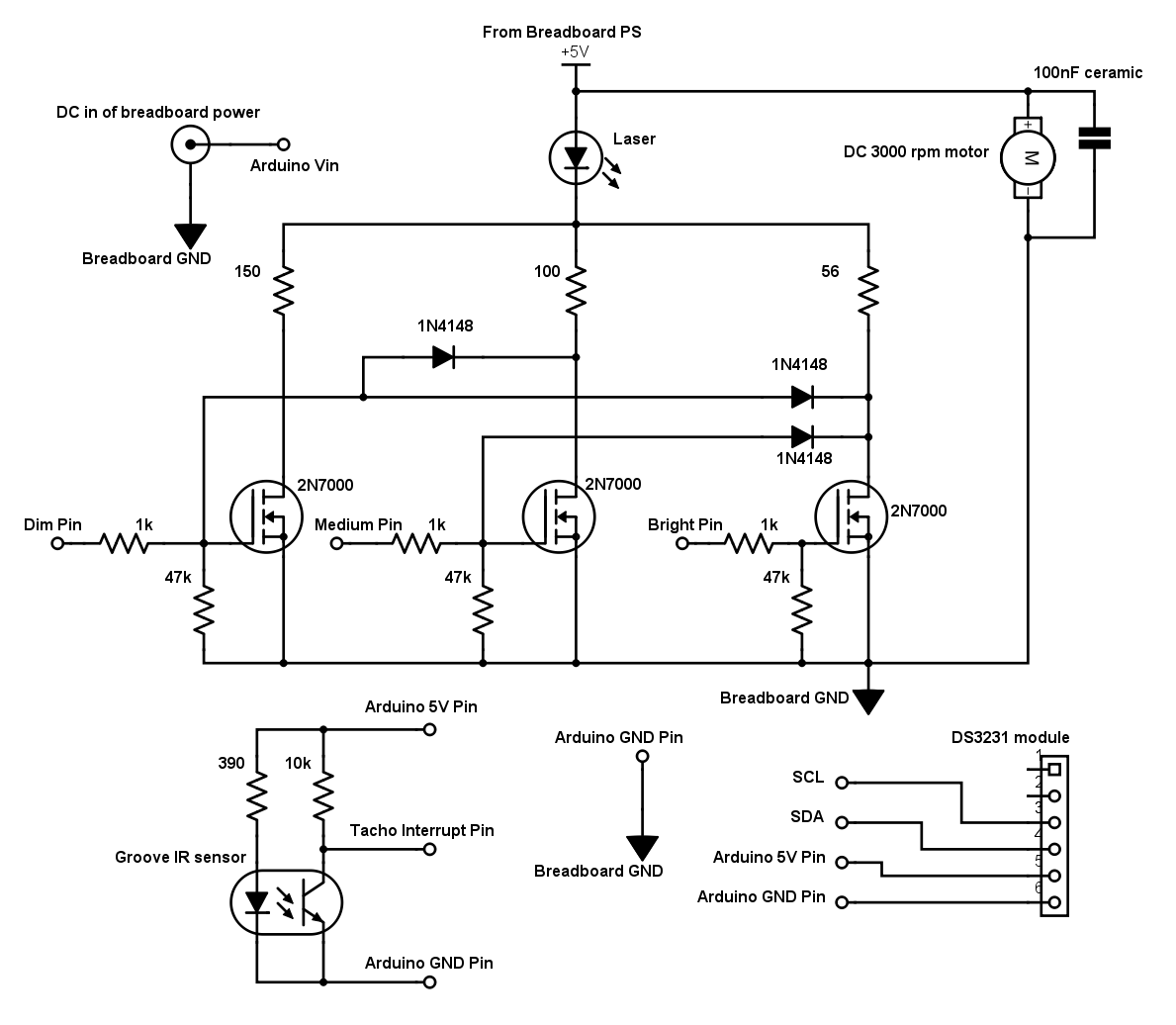# Timer Based Laser Wall Clock © GPL3+

A simple project to show a clock on a wall with just one small laser and a rotating mirror.

• 9,518 views
• 11 respects

## Components and suppliesArduino Mega 2560 Arduino Mega 1280 works as well
×1
 DS3231 RTC Module DS1307 works as well
×1
 3Volts 5mW Laser Head It's a bare component, without circuitry but with lenses
×1
 5Volts 3000 rpm DC motor Speed is somehow important but not critical (fast\powerfull motors are no good)
×1
 Pass through groove IR sensor An old PC printer will feature a pair of those, you will need just two resistors
×1×1
 2N7000 MOSFET TO92
×3
 1N4148 diode
×3
 1\4 Watt resistors See schematic to know values and quantity
×1
 Jumper wires On the market there are Male to Female, M to M and F to F... consider it
×1
 Breadboard power supply module You must solder a wire on the center pin of the DC connector to power the Arduino "Vin"
×1
 9Volts wall power supply
×1

## Necessary tools and machinesSoldering iron (generic) ALLWAYS ensure you have your iron's ground wire present and grounded or you'll blow up some ESD components
 Solder wire Don't buy it in china like this, maybe buy it at your local store, quality is important here

I don't know if someone else has come up with the same idea, but, anyway, I made it.

The project is decently simple and no requires any precision during construction (as you can see). If you are barely able to see something in the video, it's because I just don't have a nice camera; the effect is perfectly visible and neat in darkness. If you are not satisfied with light intensity, you can always use a dismissed DVD burner laser soldered on the same optics of the one I used but you will need more powerful electronics, you need a true piece of glass mirror to superglue slightly tilted on the motor pulley along with something to trigger the sensor.

### The main idea

The principle is simple: the shutter triggers the "tacho" interrupt that resets the timers, turn period is also measured, trigger points are placed along the timer's ticking, trigger points are calculated accordingly to clock's arms positions, the mirror deflects the beam around the face of the clock and the 3 transistors are triggered at timer compares obtaining different light intensities.

I left you to add a way to adjust time, for example with two buttons, the `loop()` function doesn't need to be fast.

### Some tips

• The sensor must be placed where the shutter just opens and the beam points at 12:00.
• The motor direction must make the beam spin clockwise (so if it doesn't, exchange wires).
• Hold the laser head with something thin as the laser wires and keep it not too close to the motor.
• DO NOT use the regulated 5V of the Arduino, RTC and sensor for the rest of the circuit, use different lines (this is always valid).
• Solder a jumper wire on the back of the center pin of the DC connector of the breadboard power supply to power the Arduino "Vin".
• If you are not satisfied with light intensities, you can always vary value of the 3 resistors that load the laser; you can even force it a bit.
• You must balance the rotating parts, place the shutter over a screwdriver shaft and see where it stays still. Also use a very small piece of mirror, place it in the middle and secure it well.
• The shutter has a shorter arm (that doesn't trigger) with a small counterweight.

## Code

##### Main ProgramC/C++
```/*
Arduino program for the Laser Projector Wall Clock

Copyright (C) 2016  Genny A. Carogna

This program is free software: you can redistribute it and/or modify
the Free Software Foundation, either version 3 of the License, or
(at your option) any later version.

This program is distributed in the hope that it will be useful,
but WITHOUT ANY WARRANTY; without even the implied warranty of
MERCHANTABILITY or FITNESS FOR A PARTICULAR PURPOSE.  See the
GNU General Public License for more details.

You should have received a copy of the GNU General Public License
along with this program.  If not, see <http://www.gnu.org/licenses/>.
*/

#include <I2C.h>  // you must install the "Arduino I2C Master Library" before

#define dOn PORTE=B00100000;  // PE5 pin3  // dim tracts signal (clock face)
#define dOff PORTE=0;

#define mOn PORTG=B00100000;  // PG5 pin4  // medium intensity tract signal (hours)
#define mOff PORTG=0;

#define bOn PORTH=B00001000;  // PH3 pin6  // full power (yeah!!) tracts signal (minutes and seconds)
#define bOff PORTH=0;

#define dimPin 3
#define mediumPin 4
#define brightPin 6
#define tachoPin 18

#define qTractStart 0.1  // 1/12 turn "percentage"  // quadrant
#define qTractEnd 0.9
#define hTractStart 0.3  // 1/12 turn "percentage"  // hour arm
#define hTractEnd 0.7

#define loopPeriod 150  // milliseconds

volatile unsigned int TurnPeriod = 65000;  // this stores the time necessary for an actual turn (in "16MHz /8" units)
volatile bool reverse;  // this goes "1" during 11:XX and 00:XX

volatile unsigned int ocr1a = 32000;
//volatile unsigned int ocr3a = 32000;
volatile unsigned int ocr4a = 32000;
//volatile unsigned int ocr5a = 32000;
volatile unsigned int ocr1b = 32000;
volatile unsigned int ocr3b = 32000;
volatile unsigned int ocr4b = 32000;
volatile unsigned int ocr5b = 32000;
volatile unsigned int ocr1c = 32000;
volatile unsigned int ocr3c = 32000;
volatile unsigned int ocr4c = 32000;
volatile unsigned int ocr5c = 32000;

void setup(){
pinMode(dimPin, OUTPUT);
pinMode(mediumPin, OUTPUT);
pinMode(brightPin, OUTPUT);

attachInterrupt(digitalPinToInterrupt(tachoPin), tacho, FALLING);

I2c.begin();

cli();
TCCR0A = 0;  // disabling disturbing (maybe) stuff, this disables millis(), micros() and delay()
TCCR0B = 0;  //
TIMSK0 = 0;  //

//no prescaler for timer 1
TCCR1A = 0;
TCCR1B = 0;
TCCR1C = 0;
TIMSK1 = 0;
TCNT1  = 0;
OCR1A = 65000;
OCR1B = 30000;
OCR1C = 20000;
TCCR1B |= (1 << WGM12);  // CTC enabled only for timer1
TCCR1B |= (1 << CS10);
//TIMSK1 |= (1 << OCIE1A);  // we actually don't need an interrupt on "1A"
TIMSK1 |= (1 << OCIE1B);
TIMSK1 |= (1 << OCIE1C);

// /8 prescaler for others
TCCR3A = 0;
TCCR3B = 0;
TCCR3C = 0;
TIMSK3 = 0;
TCNT3  = 0;
OCR3A = 65000;
OCR3B = 64000;
OCR3C = 63000;
TCCR3B |= (1 << CS31);
//TIMSK3 |= (1 << OCIE3A);
TIMSK3 |= (1 << OCIE3B);
TIMSK3 |= (1 << OCIE3C);

TCCR4A = 0;
TCCR4B = 0;
TCCR4C = 0;
TIMSK4 = 0;
TCNT4  = 0;
OCR4A = 65000;
OCR4B = 64000;
OCR4C = 63000;
TCCR4B |= (1 << CS41);
TIMSK4 |= (1 << OCIE4A);
TIMSK4 |= (1 << OCIE4B);
TIMSK4 |= (1 << OCIE4C);

TCCR5A = 0;
TCCR5B = 0;
TCCR5C = 0;
TIMSK5 = 0;
TCNT5  = 0;
OCR5A = 65000;
OCR5B = 64000;
OCR5C = 63000;
TCCR5B |= (1 << CS51);
//TIMSK5 |= (1 << OCIE5A);
TIMSK5 |= (1 << OCIE5B);
TIMSK5 |= (1 << OCIE5C);

sei();
}

void loop(){
// you can add stuff in loop(), like a way to set the time with buttons

for (byte i = 0; i < 100; i ++) {
delayMicroseconds(loopPeriod * 10);
}  // only delayMicroseconds() works with timer0 disabled

byte temp1 = temp0 >> 4;  // with Dallas RTCs time is stored as BCD (Binary Coded Decimal)
unsigned int seconds = (temp1 * 10) + (temp0 & B00001111);  // with Dallas RTCs time is stored as BCD (Binary Coded Decimal)

temp1 = temp0 >> 4;
unsigned int minutes = (temp1 * 10) + (temp0 & B00001111);

temp1 = temp0 >> 4;
unsigned int  hours = (temp1 * 10) + (temp0 & B00001111);
if (hours >= 12) hours -= 12;  // lets keep it in 12 hours format

if(hours == 0 || hours == 11) {
reverse = 1;  // for having a contiguous track for the hours arm we reset timer3 at 6:00 position during 11:XX and 00:XX
}
else reverse = 0;

cli();
unsigned int turnPeriod = TurnPeriod;
sei();

//A,B e C happen in reverse order (CBA)
ocr1a = (turnPeriod / 3) * 2;  // dim, end of the hour (turnPeriod / 12) * 8

//ocr3a =

ocr4a = turnPeriod / 2;  // six o'clock (used for the "reverse" case)

//ocr5a =

ocr1b = ocr1a * qTractEnd;  // dim, end of the drawn quadrant hour tract

ocr3b = (((turnPeriod / 12) * hTractEnd) + ((turnPeriod / 720) * minutes)) - (turnPeriod / 24);  // hours, end of the drawn tract
if (hours == 0) ocr3b += turnPeriod / 2;  // ((turnPeriod / 12) * 6)
else if (hours == 11) ocr3b += (turnPeriod / 12) * 5;
else ocr3b += (turnPeriod / 12) * hours;

float mRatioEnd = float(minutes + 1) / 60;
ocr4b = (turnPeriod * mRatioEnd);  // minutes, end of the drawn tract

float sRatio = float(seconds) / 60;
ocr5b = (turnPeriod * sRatio) + (turnPeriod / 80);  // seconds, end of the drawn tract  // (turnPeriod / (60 * 4)) * 3

ocr1c = ocr1a * qTractStart;  // dim, start of the drawn quadrant hour tract

ocr3c = (((turnPeriod / 12) * hTractStart) + ((turnPeriod / 720) * minutes)) - (turnPeriod / 24);  // hours, start of the drawn tract
if (hours == 0) ocr3c += turnPeriod / 2;  // ((turnPeriod / 12) * 6)
else if (hours == 11) ocr3c += (turnPeriod / 12) * 5;
else ocr3c += (turnPeriod / 12) * hours;

float mRatioStart = float(minutes) / 60;
ocr4c = turnPeriod * mRatioStart;  // minutes, start of the drawn tract
if (ocr4c < 100) ocr4c = 100;  // lets try to NOT trigger a compare exactly when a timer resets and lets the "tacho" do it's job

ocr5c = (turnPeriod * sRatio) + (turnPeriod / 240);  // seconds, start of the drawn tract  // turnPeriod / (60 * 4)
}

void tacho() {
dOff
bOff

OCR1A = ocr1a;  // refresh trigger points on various timers
//OCR3A = ocr3a;  //
OCR4A = ocr4a;  //
//OCR5A = ocr5a;  //
OCR1B = ocr1b;  //
OCR3B = ocr3b;  //
OCR4B = ocr4b;  //
OCR5B = ocr5b;  //
OCR1C = ocr1c;  //
OCR3C = ocr3c;  //
OCR4C = ocr4c;  //
OCR5C = ocr5c;  //

TurnPeriod = TCNT4;  // read motor speed for calculations

TCNT1 = 0;  // restart timers
TCNT4 = 0;  //
TCNT5 = 0;  //
if (!reverse) {
TCNT3 = 0;  // this in case we are NOT at 11:XX or 00:XX
mOff
}
}

/*
ISR(TIMER1_COMPA_vect){

}
*/
ISR(TIMER1_COMPB_vect){
dOff  // comment this for reversed quadrant picture (small tracts at "o'clocks" VS. big tracts into the hour span (default))
//dOn  // uncomment this for reversed quadrant picture
}

ISR(TIMER1_COMPC_vect){
dOn  // comment this for reversed quadrant picture
//dOff  // uncomment this for reversed quadrant picture
}

/*
ISR(TIMER3_COMPA_vect){

}
*/
ISR(TIMER3_COMPB_vect){
mOff  // hour, end of the tract
}

ISR(TIMER3_COMPC_vect){
mOn  // hour, start of the tract
}

ISR(TIMER4_COMPA_vect){
if (reverse) {  // this in case we ARE at 11:XX or 00:XX
TCNT3 = 0;
mOff
}
}

ISR(TIMER4_COMPB_vect){
bOff  // minutes, end of the tract
}

ISR(TIMER4_COMPC_vect){
bOn  // minutes, start of the tract
}

/*
ISR(TIMER5_COMPA_vect){

}
*/
ISR(TIMER5_COMPB_vect){
bOff  // seconds, end of the tract
}

ISR(TIMER5_COMPC_vect){
bOn  // seconds, start of the tract
}
```
##### Program For Reading Motor Speed (can be usefull)C/C++
```/////////////////////////////////////////////////////////////////////////////////////////////////////////////////////////////////////////
//Sketch for reading motor speed, pin18 is intended for the Arduino Mega, value displayed on serial monitor is microseconds period
//for one turn so if it's 19500 it means 0.0195 seconds and it means (1/X) ~50Hz and it means (X * 60) 3000 rpm (revolutions per minute)

#define interruptPin 18  // intended for the Mega

volatile unsigned long j = 0;

void setup() {
Serial.begin(9600);
attachInterrupt(digitalPinToInterrupt(interruptPin), tacho, FALLING);
}

void loop() {
delay(500);
Serial.println(j);
}

void tacho() {
static unsigned long i;
j = micros() - i;
i = micros();
}
```
```////////////////////////////////////////////////////////////////////////////////////////////////////////////////////////////////////////////////////
//Sketch for uploading time on the RTC chip (adjust this sketch with 5 minutes more -> burn it -> disconnect the Arduino power
//-> stick battery on the module -> connect the RTC to the board -> plug the USB at the exact time and open serial monitor)(don't connect it again)

#include <Wire.h>

void setup() {
Serial.begin(9600);
Wire.begin();

Wire.beginTransmission(0x68);  // address of the RTC (do not modify)
Wire.write(byte(0x00));  // address of the first data byte (seconds) (do not modify)

Wire.write(byte(0x00));  // seconds (from 0x00 to 0x59)
Wire.write(byte(0x30));  // minutes (from 0x00 to 0x59)
Wire.write(byte(0x10));  // hours (from 0x00 to 0x23)
Wire.write(byte(0x04));  // day of the week (from 0x01 to 0x07)(this is arbitrary)
Wire.write(byte(0x26));  // day of the month (from 0x01 to 0x31)(this is NOT arbitrary)
Wire.write(byte(0x05));  // month (from 0x01 to 0x12)
Wire.write(byte(0x16));  // year (from 0x00 to 0x99)

Wire.endTransmission();
}

void loop() {
delay(1000);

Wire.beginTransmission(0x68);
Wire.write(byte(0x00));
Wire.endTransmission();

Wire.requestFrom(0x68, 7);

Serial.print("Right now it's: ");
Serial.print(hours, HEX);
Serial.print(":");
Serial.print(minutes, HEX);
Serial.print(":");
Serial.println(seconds, HEX);

Serial.print("Today is day ");
Serial.print(dayWeek);
Serial.println(" of the week");

Serial.print("Current date is: ");
Serial.print(dayMonth, HEX);
Serial.print("/");
Serial.print(month, HEX);
Serial.print("/20");
Serial.println(year, HEX);
Serial.println();
}
```
##### Arduino I2C Master Library
It's an alternative to the Wire library that doesn't rely on interrupts (useful to use inside an ISR, the Wire library CANNOT run inside an ISR), GIthub has the initial release, consider taking the latter from their website.

## SchematicsMay 28, 2016

#### Members who respect this project

See similar projects
you might like

#### Animated RGB Wall Clock

Project tutorial by TheTNR

• 21,872 views
• 46 respects

#### Android Things Word Clock

Project tutorial by Daniele Bonaldo

• 33,295 views
• 152 respects

#### Digital Wall Clock

Project tutorial by Deep_Sky

• 16,691 views
• 35 respects

#### 3D Printed Lunar Phase Clock

Project tutorial by G4lile0

• 9,892 views
• 62 respects

#### RTC Based Clock

Project tutorial by shivakumarj1995

• 20,085 views